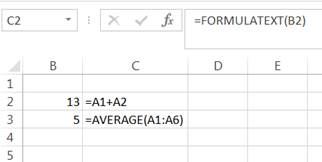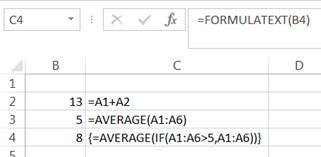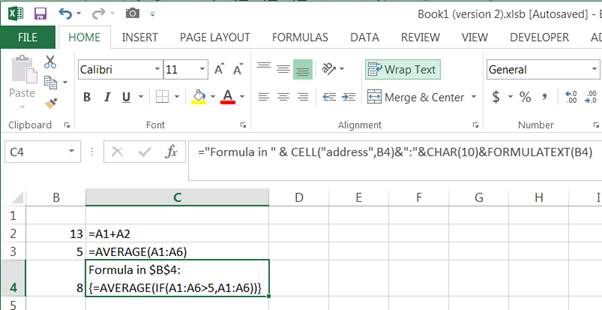Home > Publications & Training > Case Studies > The Excel FORMULATEXT functionShare Your

# FORMULATEXT function

With Excel 2013, Microsoft introduced several new functions, one of which is worth a separate mention. FORMULATEXT displays as text the formula in a cell.

Suppose cell B2 contains the formula =A1+A2 and cell B3 the formula =AVERAGE(A1:A6).

To display the formulas in adjacent cells (cells C2 and C3), use =FORMULATEXT(B2) and =FORMULATEXT(B3) respectively as in Figure 1.## FORMULATEXT and array formulas

FORMULATEXT shows an array formula enclosed in curly brackets { and } – just as it would appear in the formula bar. See Figure 2.## Improving the display of FORMULATEXT

We can improve how the formula is shown by using FORMULATEXT as part of a larger formula.  For example, in Figure 3 the result of the formula in C4 is more comprehensive than in Figure 2.  The use of CHAR(10) and turning on Wrap Text yields an easy to read result in C4.